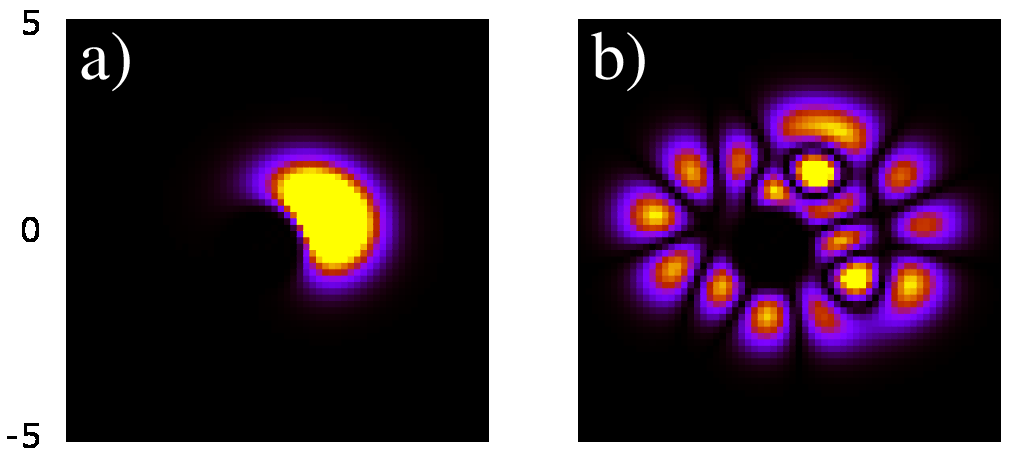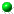Dynamics and thermalization
of Bose-Einstein condensate
in Sinai oscillator trap
by L.Ermann, E.Vergini and D.L.Shepelyansky
arXiv:1605.02831[cond-mat.quant-gas]Quantum probability in (x,y) plane: a) ground state of Sinai oscillator, b) initial exited state m=24, e)steady state at time t=2000, f)theoretical Bose-Einstein distribution; here nonlinearity beta=4

 ` `Article download arXiv-v1 (May 2016): (here)All figs in gzip format (here )First 100 linear eigenstates in x,y-plane (color shows wavefunction probability; format first100egv.flv) (video)First 100 linear eigenstates in momentum representation p_x,p_y-plane (color shows wavefunction probability; format first100egvvel.flv) (video)First 100 linear eigenstates in x,y-plane for integrable case without disk (color shows wavefunction probability; format first100egv.integrable.flv) (video) ; momentum representation p_x,p_y-plane (format: first100egv.mom.integrable.flv; video) ;Video of time evolution from t=0 to t=2500 of initial state m=24, beta=4 (color shows wavefunction probability; format animi24b4.flv ) (video)Video of time evolution from t=0 to t=5000 of initial state m=24, beta=4 (color shows wavefunction probability; format animlongi24b4.flv ) (video)Video of time evolution from t=0 to t=100 of initial coherent state, beta=0 (color shows wavefunction probability; format animicohx3y3b0.flv ) (video)Video of time evolution from t=0 to t=100 of initial coherent state, beta=4 (color shows wavefunction probability; format animicohx3y3b4.flv ) (video)Video of time evolution from t=0 to t=5000 of initial coherent state, beta=4 (color shows wavefunction probability; format animlongicohx3y3b4.flv ) (video)Related research on GPE in Bunimovich stadium L.Ermann, E.Vergini, D.L.Shepelyansky EPL v.111, p. 50009 (2015) (web site)Related research on dynamical thermalization in nonlinear chains L.Ermann, D.L.Shepelyansky New. J. Phys. v.15, p.123004 (2013) (paper; video abstract) This webpage is created at May 9, 2016 and is maintained by L.Ermann and D.L.Shepelyansky